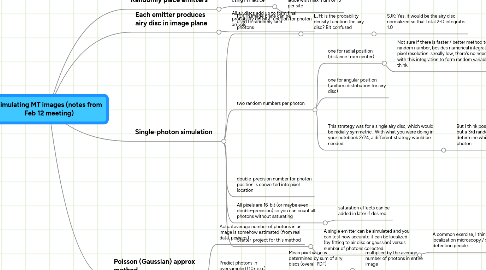# Simulating MT images (notes from Feb 12 meeting)

Get Started. It's FreeSimulating MT images (notes from Feb 12 meeting)## 1. Randomly place emitters

### 1.1. using MT lattice

1.1.1. Simple method is 8 nm latice with maximum of 13 per site

## 3. Single-photon simulation

### 3.1. PDF in image plane can be used to randomly land photons

3.1.1. LJH: is the probability density function the airy disc? Bit confused

3.1.1.1. SJK: Yes, it would be the airy disc normalized so that total 2-D integral is 1.0

### 3.2. two random numbers per photon

3.2.1. one for radial position (distance from center)

3.2.1.1. Not sure if there is faster / better method to get this random number, besides numerical integration. Since pixel resolution is really low, there's no need to be super-accurate with this integration to form random variable, I think.

3.2.2. one for angular position (uniform distribution for airy disc)

3.2.3. This strategy was for a single airy disc, which would be radially symmetric. With what you were doing in your notebook 2/24, a different strategy would be needed.

3.2.3.1. But I think possibly you can still do this method, but a 3rd random number is needed to determine which of the emitters emitted the photon.

3.2.3.1.1. Random #1--which emitter?

3.2.3.1.2. Random #2--what angle around the emitter? (phi)

3.2.3.1.3. Random #3--how far from emitter? (r)

### 3.4. All pixels are 16-bit (or maybe even double-precision) so you can count all photons without saturating

3.4.1. saturation effects can be added in later if desired.

### 3.5. Starter-project for this method

3.5.1. A single emitter can be simulated and you can test how accurate it can be localized (by fitting to air disc or gaussian) versus number of photons collected.

3.5.1.1. A common exercise, I think for localization microscopy / single-molecule detection people.

## 4. Poisson (Gaussian) approx method

### 4.2. Predict photons in oversampled (10x e.g.) image

4.2.1. Mean pixel value is determined by sum of airy discs (overall PDF)

4.2.1.1. multiplied by the average number of photons in entire image

4.2.2. noise is determined by poisson statistics